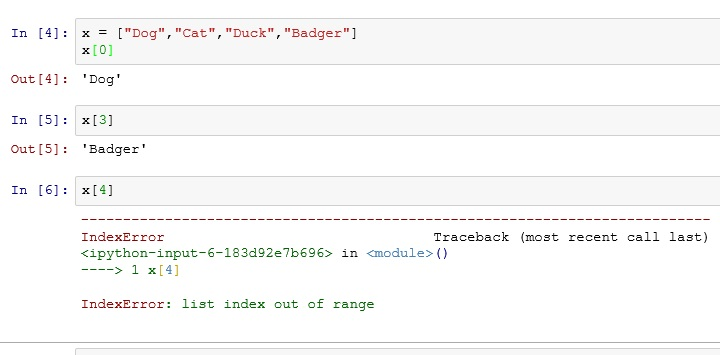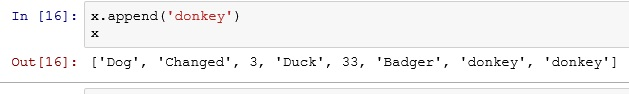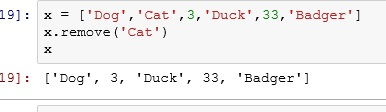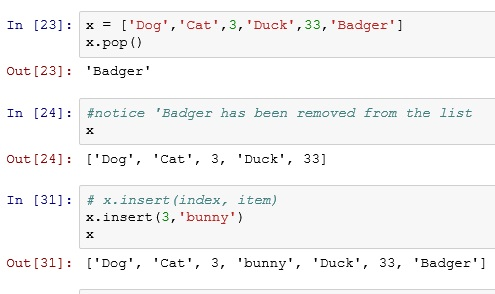# Python: Working with Lists

Making a list in Python is simple: x = [1,2,3,4]

Now let us see how we can work with them.

### Index

First thing to understand is that a list is indexed. Each item in the list is given a number that tells you its position in the list.

It is important to note that Python is a 0 index language. This means indexes begin at 0 not 1. So the first item in the list, is found by calling: xNote that x returns an error. Since there are 4 items in the list, the indexes go 0,1,2,3. Index 4 is out of range.

Another interesting point to understand about indexes is that you can use a negative index. x[-2]  returns Duck

### Index Range

Use a “:” to return a range of items from a list: x[start:end]. If you leave out the start or end number, the index starts or ends at the start or end of the list### Update Values in a List

If you want to change a value in a list, just assign it a new value like you would with a regular variable.### Append()

If you want to add an item to then end of a list, you can use the Append() function### Del

To delete a item from a list, use the Del command.### Remove()

Remove works like Del, except instead of using index values, Remove() uses the values stored in the list.### Pop() and Insert()

Pop() simply returns the last item from a list

Insert() lets you add a item to a list, but it also lets you choose what position in the list to add it.### Len()

Len() returns a count of the number of items in a list.If you enjoyed this lesson, click LIKE below, or even better, leave me a COMMENT.

Last Lesson: Python Lists and Dictionaries

Next Lesson: Working with Dictionaries

Back to Python Course: Course